# Logarithms

The logarithm is the inverse operation of exponentiation. It is used to do calculations without any calculating device. The calculating machines are failed in some cases even though we are in a very advanced stage technically but logarithms never fail. So, it is essential for everyone to learn logarithmic mathematics.

## Basics

There are three basic mathematical concepts to start learning the logarithms in mathematics. So, let’s learn each of them to begin studying the logarithms.

#### ExponentsLearn the concept of the exponents from basics to advanced level with problems.

#### Introduction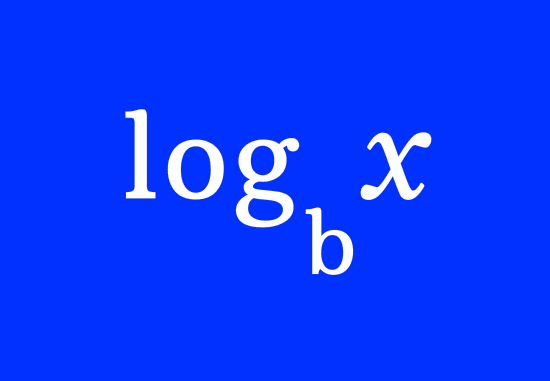Introduction to the logarithm to learn how to express the quantities in logarithms.

#### RelationshipLearn the mathematical relationship between exponents and logarithms.

### Systems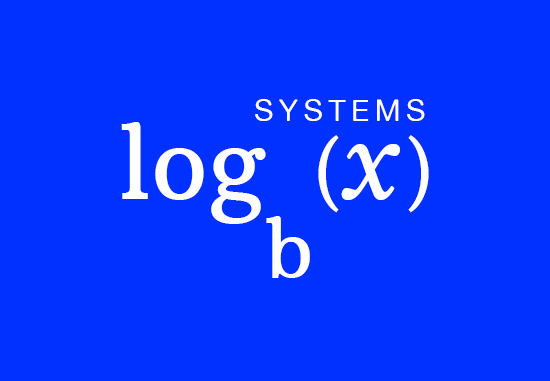Learn how to define a logarithmic system with understandable examples and a list of some popular logarithmic systems.

### Intermediate Level

There are three more concepts to upgrade your knowledge on logarithms to the next level. Now, let’s learn each of them with understandable examples.

#### Terms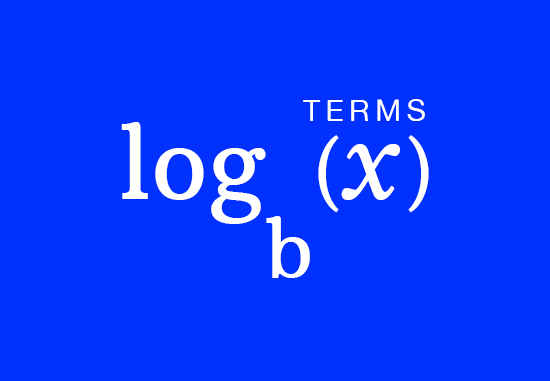Learn how to express the quantities in the form of logarithmic terms.

#### Operations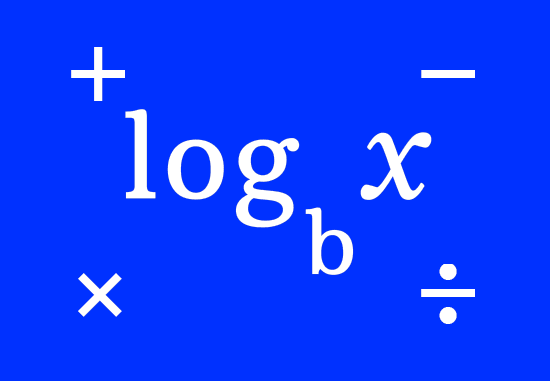Learn how to perform the basic mathematical operations with log terms.

#### Identities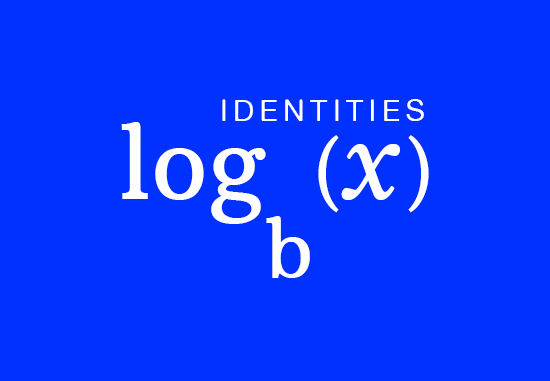Learn the mathematical relationship between exponents and logarithms.

Learn how to use your mathematical knowledge on the logarithms in mathematics.

#### Expressions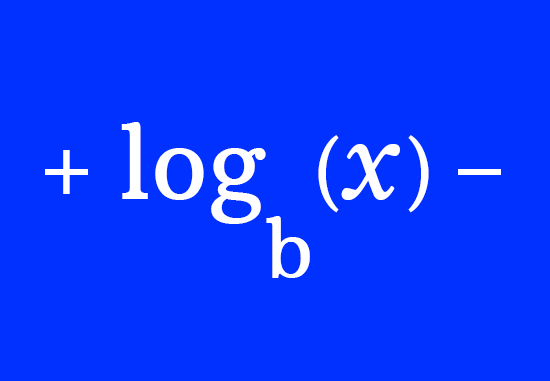Learn how to simplify the logarithmic expressions to evaluate them.

#### Equations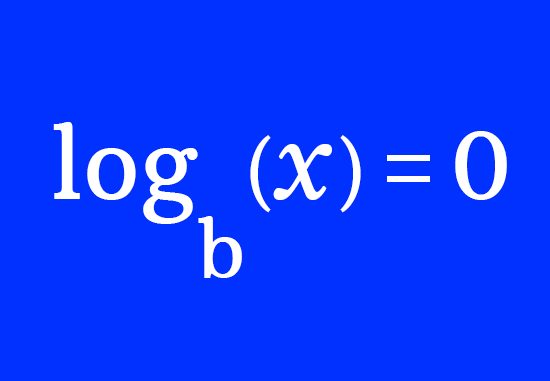Learn how to solve the logarithmic equations for finding the variables.

#### Inequations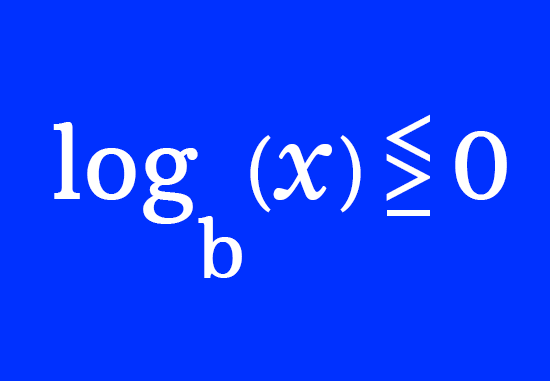Learn how to solve the logarithmic inequations to evaluate the variables.

###### Math Questions

The math problems with solutions to learn how to solve a problem.

Learn solutions

Practice now

###### Math Videos

The math videos tutorials with visual graphics to learn every concept.

Watch now

###### Subscribe us

Get the latest math updates from the Math Doubts by subscribing us.